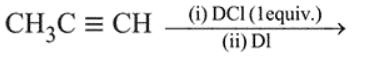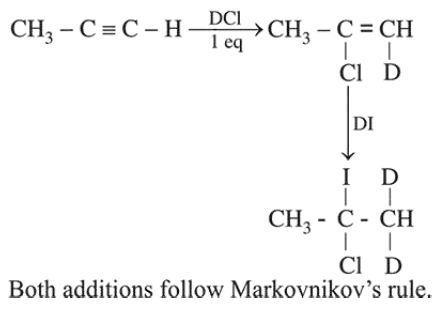# The major product of the following reaction is:Question:

The major product of the following reaction is:1. $\mathrm{CH}_{3} \mathrm{CD}(\mathrm{I}) \mathrm{CHD}(\mathrm{Cl})$

2. $\mathrm{CH}_{3} \mathrm{CD}(\mathrm{Cl}) \mathrm{CHD}$ (I)

3.  $\mathrm{CH}_{3} \mathrm{CD}_{2} \mathrm{CH}(\mathrm{Cl})$ (I)

4. $\mathrm{CH}_{3} \mathrm{C}(\mathrm{I})(\mathrm{Cl}) \mathrm{CHD}_{2}$

Correct Option: , 4

Solution: